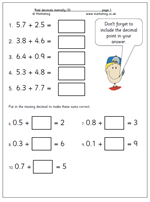Here is another page which looks at adding decimals mentally and once again shows the different approach which is usually taken compared to the standard written method.

To illustrate what I mean let’s look at the first question: 5.7 + 2.5. If this was done by the written method the two numbers would be placed in a vertical form:

5.7

2.5

and added starting with the tenths before going on to the units.

When using mental methods it would be quite usual to start with the units, adding the 5 and 2 to make 7, holding this in our memory, then adding the 7 and 5 tenths, making 1.2 before finally adding the 1.2and 7 to make 8.2.

The second set of questions on the page are probably best done by adding on. For example:

0.8 + ?? = 3

I would do this by adding 0.2 to 0.8 to make 1 and then counting on 2 more to make 3. There are , of course, other ways but the important thing is that whatever method is used it needs to be quick and accurate.

More of this type of question can be found in our Four Rules category.

## Division: 3-digit numbers divided by 8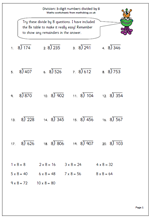Here is another page for practising the short division method, this time just using the eight times table. The key to this method is, of course, a good knowledge of the 8 times table, as without this the solution can take an awfully long time and lots of errors may occur. The table has been written out as a helpful starting point, so that the method can be concentrated on.

For example: 8)659

‘How many eights in 65?’

‘8 times 8 is 64, 9 times 8 is 72 which is too much.’

‘8 goes into 65, 8 times with 1 left over.’

Write the 8 in the answer above the 5.

Write the remainder 1 beside the 9 units, making 19.

‘How many eights in 19?’

‘2 times 8 is 16.’

‘8 goes into 19, 2 times with 3 left over.’

Write the 2 in the answer in the units and write rem 3 next to it.

Division: 3 digits by 8

## More 2x, 5x and 10x tables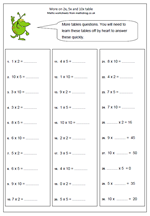Children need an awful lot of practice when learning the 2x, 5x and 10x tables and don’t be afraid to encourage them to say these tables out loud and learn them by rote. You will probably find that they can recite the lyrics of a latest pop song, or all eleven of their favourite football team’s line up so there is little or no reason why they can’t learn tables – motivation is probably the main factor.

This page will show immediately whether these tables have been learned. It can be answered very quickly – just a couple of minutes, or it may take some time. Anything over 3 or 4 minutes will show that the times tables have not been learned off by heart.

More 2x, 5x, 10x practice

## Resource of the Week: written subtraction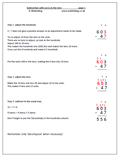This week I am looking at one of the hardest ideas to get across to children; carrying out a subtraction which requires adjusting across two columns. This only happens when there is a zero involved.

For example:

703

286 –

3 – 6 does not give a positive answer so an adjustment to the top number needs to be made. A ten can not be borrowed as there are none.

The procedure then is to borrow from the hundreds, move to the tens and then move again to the units. Often children will borrow from the hundreds, ignore the zero in the tens and move straight to the units. They cross out the 7 and make it 6 and make the 3 into 13.

This does not work as it has been 100 borrowed, not 10. Extra steps need to be taken by making the tens column 10 and then taking one ten, making this 9, and adjusting to the units.

Some children will see a quicker way: making the 70 one less (69) and adjusting to the units.

More explanation is available on the worksheet.

Standard subtraction with zeros in the tens (pg 1)

## Long multiplication: 3 by 2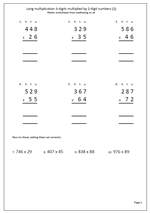I am often asked if there are more basic number sheets in the pipeline and so as to not disappoint people here is another page of long multiplication. The first six questions have been laid out in the correct way but the last four have been put in a horizontal position and will need laying out correctly.

The key with multiplying by 2-digit numbers is to multiply by the tens and then the units and finally add the two answers together. Some prefer to multiply by the units first: it makes no difference. Of course when multiplying by the tens digit it is important to remember to place a zero in the units column, ensuring that all other digits are then placed one place to the left and have a value ten times more.

It is also important to keep the numbers in straight columns to make adding up easier.

Long multiplication 3 by 2 (2)

## Short multiplication by 2, 5 and 10.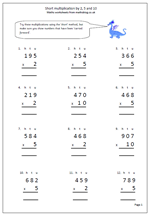When children are learning the standard methods of multiplication they need plenty of practice. With multiplication there are two distinct methods, the long and the short! Most schools tend to teach the long method first as it is clearer to show exactly what is happening, but it is also important for children to learn the short method, as we are looking to achieve fast accurate calculating.

This page keeps it simple by just multiplying by 2, 5 and 10; three tables that children should know ‘off by heart’.The questions have been laid out in the correct way so all that has to be done is:

a. Firstly to multiply by the units, remembering to carry any tens across to the tens column. These are usually placed under the answer.

b. Secondly multiply the tens remembering to carry any hundreds across to the hundreds column

c. Finally multiply the hundreds and write in the answer, including any thousands.

Short multiplication by 2, 5 and 10

## 2 times table up to 12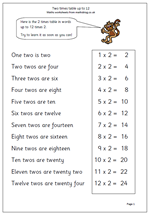Learning times tables off by heart is a fundamental part of primary maths, yet many children go on to High School without knowing them. This really inhibits progress with a whole range of other maths, including long and short  multiplication, long and short division, factors, multiples etc.

There are not that many facts to learn and once learned off by heart they are seldom forgotten.

Here are a couple of starter pages for the two times table. I have gone up to 12 x 2 because the new national Curriculum for maths seems to have this as one of its requirements next year, although many people would argue that learning tables up to 10 covers all necessary requirements.

Thanks to urbrainy.com for letting me use this resource from their wide range of worksheets.

2x table up to 12

## Written method for adding 4-digit numbers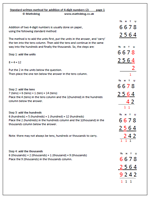Addition of two 4-digit numbers is usually done on paper,using the following standard method:

The method is to add the units first, put the units in the answer, and ‘carry’ the ten into the tens column. Then add the tens and continue in the same way into the hundreds and finally the thousands. So, looking at the addition of 6678 + 2564,

the steps are:

8 + 4 = 12

Put the 2 in the units below the question.
Then place the one ten below the answer in the tens column.
7 (tens) + 6 (tens) + 1 (ten) = 14 (tens)
Place the 4 (tens) in the tens column and the 1(hundred) in the hundreds column below the answer.

6 (hundreds) + 5 (hundreds) + 1 (hundred) = 12 (hundreds)
Place the 2 (hundreds) in the hundreds column and the 1(thousand) in the thousands column below the answer.

Note: there may not always be tens, hundreds or thousands to carry.

6 (thousands) + 2 (thousands) + 1 (thousand) = 9 (thousands)
Place the 9 (thousands) in the thousands column.

More clarification is available on the worksheet, together with a set of additions laid out in the correct format.

Standard addition of 4 digit numbers (2)

## Divide 3-digit numbers by 7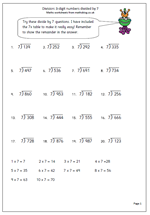Here is another page for practising the short division method. When I was young I was taught the short method before the long method and by repeated practice learned the method, although probably not knowing why it works. Today the long method is usually introduced first as a means of showing exactly how it works, usually with easier numbers. The key to both methods is, of course, a good knowledge of tables, although on this page I have written out the 7 times table to help.

For example: 7)627

‘How many sevens in 62?’

‘7 times 8 is 56, 7 times 9 is 63.’

‘7 goes into 62, 8 times with 6 left over.’

Write the 8 in the answer above the 2.

Write the remainder 6 beside the 7 units, making 67.

‘How many sevens in 67?’

‘9 times 6 is 63.’

‘7 goes into 67, 9 times with 4 left over.’

Write the 9 in the answer in the units and write rem 4 next to it.

Division 3-digit numbers by 7

## Short multiplication by 6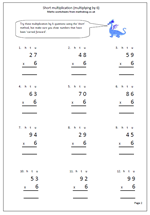Here is the latest worksheet which looks at the short multiplication method; this time multiplying 2-digit numbers by 6. It is called the short method because it eliminates the process of putting parts of the answer in two or three columns below, as in the ‘long’ method. The stages are the same as before:

1. writing the question out correctly so that it is in vertical format. it is a good idea to write a small h, t and u above to show the hundreds, tens and units columns.

2. Multiplying the units and carrying any of the tens across, below the answer line.

3. multiplying the tens and putting any hundreds into the answer.

More clarification of this method is shown on the worksheet.

The 6 times table is one of the harder tables to learn, but knowing it off by heart makes this type of multiplication so much easier.

Short multiplication by 6